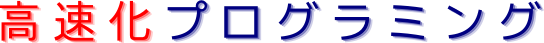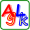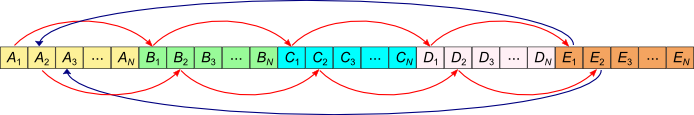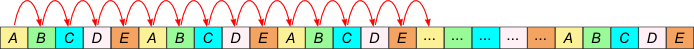# 構造体の配列: Sample 2: 構造体のメンバーの和の計算(C)トップ  >  メモリジャンプと高速化  >  構造体の配列  >  Sample 2: 構造体のメンバーの和の計算(C)

## 構造体の配列: Sample 2: 構造体のメンバーの和の計算(C)

### ■ 概要

ここでは構造体の全部のメンバーの和を別の配列にコピーするサンプルを示します。内容的にはSample 1と似ていますが、違いは配列rに代入するのはあるひとつのメンバーではなくて、全部のメンバーの和です。つまりSample 1ではひつとのメンバーだけにアクセスしますが、このサンプルでは全メンバーにアクセスします。

### ■ ソースコード

 ◆ Code 1 ◆ Code 2 ``` ``` // Program to take the sum of the members of a structure to another array #include #include struct Vector { double *a, *b, *c, *d, *e, *f, *g, *h; }; int main() { int i, k, n; Vector vec; double *r; printf("Array size Elapsed time [sec] \n"); for (int k=1; k<=20; k++) { // Array size int n = k * 2000000; // Allocation r = new double[n]; vec.a = new double[n]; vec.b = new double[n]; vec.c = new double[n]; vec.d = new double[n]; vec.e = new double[n]; vec.f = new double[n]; vec.g = new double[n]; vec.h = new double[n]; // Initialization for (i=0; i #include struct Vector { double a, b, c, d, e, f, g, h; }; int main() { int i, k, n; Vector *vec; double *r; printf("Array size Elapsed time [sec] \n"); for (int k=1; k<=20; k++) { // Array size int n = k * 2000000; // Allocation r = new double[n]; vec = new Vector[n]; // Initialization for (i=0; i

### ■ 計算時間の測定結果

Code 1Code 2を実行したときの計算時間をそれぞれ青線と赤線で図1に示します。そして両者の比を緑線で示します。### ■ 考察

Code 1の配列の構造体では配列のメンバーが連続的に配置されているので、構造体の全部のメンバーを順番ににアクセスするとメモリのジャンプが起こります(図2を参照)。一方、Code 2の構造体の配列では構造体の同じメンバーは不連続に配列されているので、このサンプルでは図3のようにメモリのジャンプが起こりません。## その他

 4 5 4 8 7 8